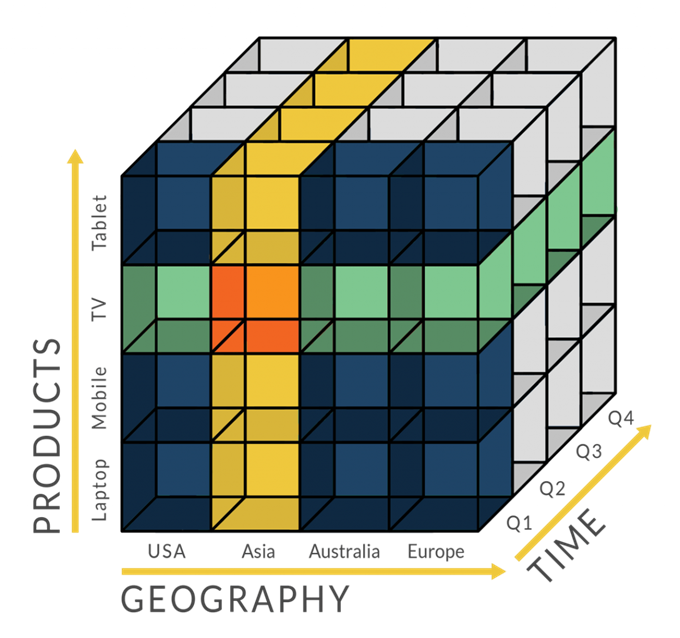# Pandas中DataFrame的合并与连接

import pandas as pd
import glob

file_list = glob.glob('data/*')
df_list = []
for file in file_list:
df_temp = pd.read_csv(file, sep="import pandas as pd
import glob
file_list = glob.glob('data/*')
df_list = []
for file in file_list:
df_list.append(df_temp)
df = pd.concat(df_list, ignore_index=True)
df_list.append(df_temp)
df = pd.concat(df_list, ignore_index=True)


Pandas中合并数据的方法总共有4个：

• append()
• concat()
• merge()或df.merge()
• join()

## df.append()

df.append()比较好理解，就是在原有的数据中追加数据，语法为：df1.append(df2, sort=False)，效果类似SQL中的UNION ALL。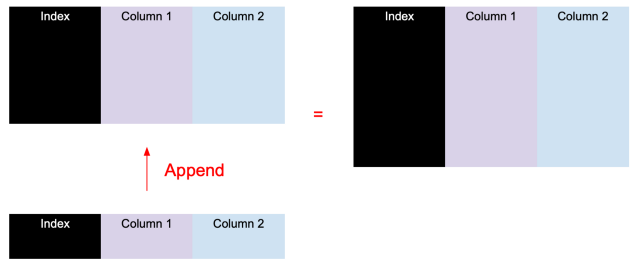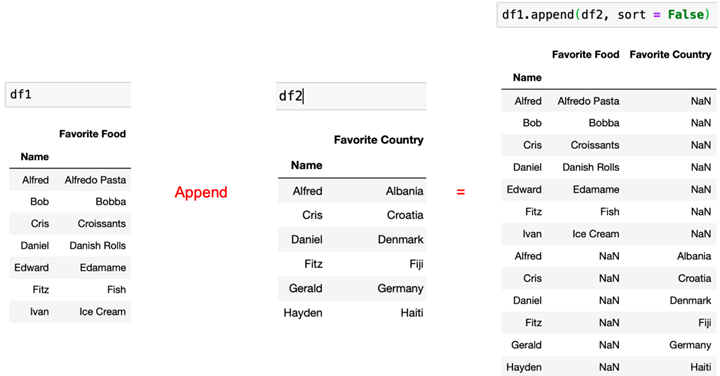## pd.concat()

pd.concat() 相当于df.append()的加强版本。它除了支持合并多个DataFrame外，除了垂直（纵向）合并，还支持水平（横向）合并。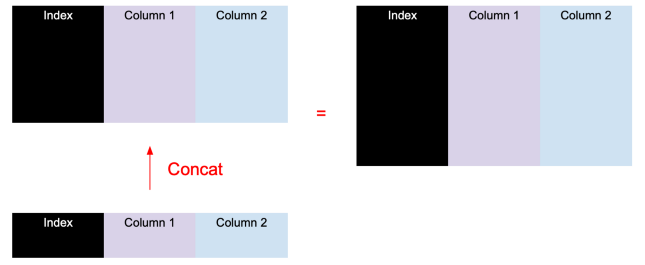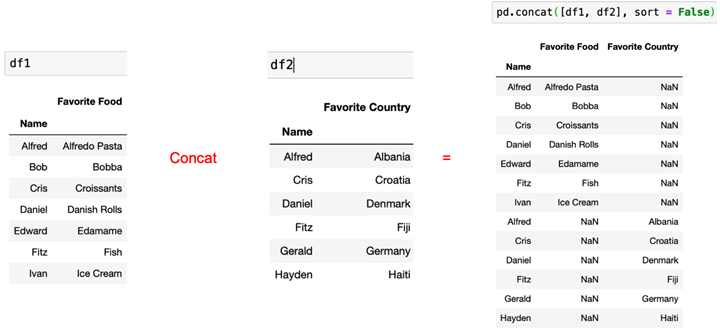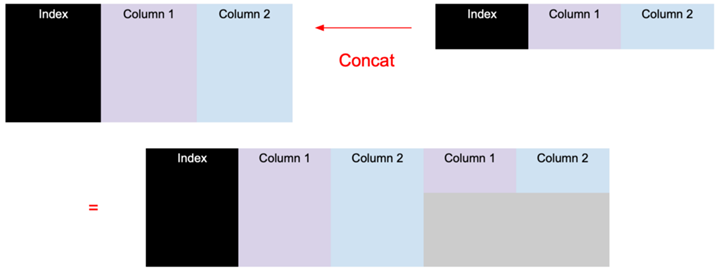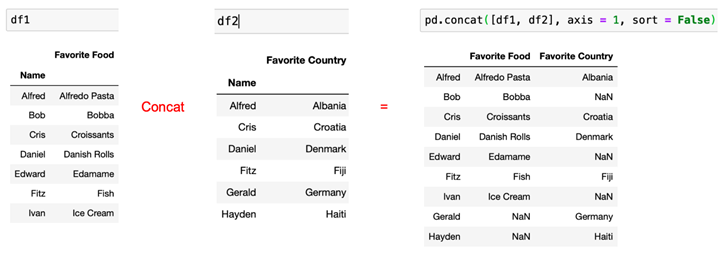pandas.concat(objs, axis=0, join=’outer’, ignore_index=False, keys=None, levels=None, names=None, verify_integrity=False, sort=False, copy=True)

• objs：要连接的 Pandas Series 或 DataFrame 对象的序列或映射。
• join：连接方法(inner 或 outer)
• axis：沿着行(axis=0)或列(axis=1)进行连接
• ignore_index：如果为 True，则忽略原 DataFrames 的索引。
• keys：向结果索引添加标识符的顺序
• levels：用于创建 MultiIndex 的级别
• names：多重索引中的级别名称
• verify_integrity：布尔型。如果为 True，则检查是否有重复。
• sort：布尔型。当 join 为 outer 时，如果非 concatenation 轴尚未对齐，则对其进行排序。
• copy：布尔型。如果为 False，避免不必要的数据复制。

## pd.merge()或df.merge()

pandas提供了一个类似于关系数据库的连接(join)操作的方法merage,可以根据一个或多个键将不同DataFrame中的行连接起来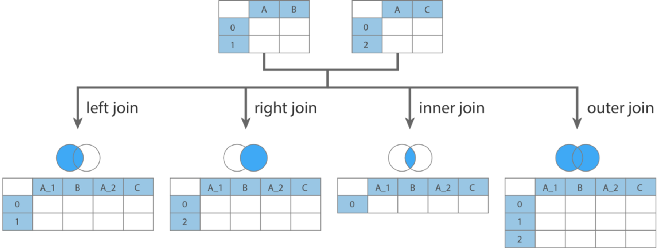DataFrame.merge(right, how=’inner’, on=None, left_on=None, right_on=None, left_index=False, right_index=False, sort=False, suffixes=(‘_x’, ‘_y’), copy=True, indicator=False, validate=None)

• right：连接时右侧的DataFrame
• how：连接方式，可选值{‘left’, ‘right’, ‘outer’, ‘inner’, ‘cross’}，默认‘inner’
• left：类似SQL的left join
• right：类似SQL的right join
• outer：类似SQL的full join（全连接）
• inner：类似SQL的inner join
• cross：类似SQL的cross join(笛卡尔乘积)
• on：类似SQL的on，要求左右两个DataFrame具有相同的列名。
• left_on：左Dataframe关联的列名
• right_on：右Dataframe关联的列名，可以与左边的名称不一样。
• left_index：使用左侧DataFrame中的行索引作为连接键；
• right_index：使用右侧DataFrame中的行索引作为连接键；
• sort：默认为True，将合并的数据进行排序，设置为False可以提高性能；
• suffixes：字符串值组成的元组，用于指定当左右DataFrame存在相同列名时在列名后面附加的后缀名称，默认为(‘_x’, ‘_y’)；
• copy：默认为True，总是将数据复制到数据结构中，设置为False可以提高性能；
• indicator：显示合并数据中数据的来源情况
• validate：验证选项
• “one_to_one” or “1:1”: 确定是否左表与右表是否是一对一
• “one_to_many” or “1:m”: 确定是否左表与右表是否是一对多
• “many_to_one” or “m:1”: 确定是否左表与右表是否是多对一
• “many_to_many” or “m:m”: 确定是否左表与右表是否是多对多

## df.join()

df.join()是dataframe内置的join方法，默认以index作为对齐的列。功能相对于merge()弱，这里就不做详细介绍了。

DataFrame.join(other, on=None, how=’left’, lsuffix=”, rsuffix=”, sort=False)

• other：右侧的DataFrame
• on：同上
• how：同上
• lsuffix：左DataFrame中重复列的后缀
• rsuffix：右DataFrame中重复列的后缀
• sort：同上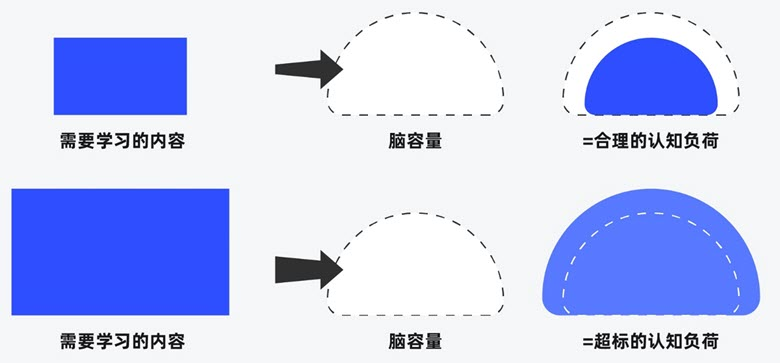##### 界面设计和游戏设计中的认知负荷##### 理解认知偏差